Health Care Journals | Open Access - Edelweiss | Edelweiss Publications

Magnetic Field Effects on Aqueous Anionic and Cationic Surfactant Solutions Part II: Surface Tension

Emil Chibowski, Aleksandra Szcześ and Lucyna Hołysz

DOI Number: https://doi.org/10.33805/2641-7383.107PDF

Abstract

Magnetic Field (MF) effects on water and dispersed systems have been studied for more than half of century. However, so far there is no complete understanding of the observed changes in the properties of studied systems if it is solely based on the classical theories of magnetic field action. In Part I of this paper we presented results of MF influence on the evaporation rate of aqueous solutions of anionic surfactant Sodium Dodecyl Sulphate (SDS) and cationic one Dodecyl Trimethylammonium Bromide (DoTAB). In this paper static magnetic field effects on the surface tension of the above-mentioned surfactants are presented. The concentrations of the solutions used were both below and above their Critical Micelle Concentration (CMC). The solutions were under the MF action for 60 min in a closed vessel and were stirred by hand every 15 min. Previously it was found that the surface tension of pure water (distilled in a quartz apparatus) decreased by 2.1 mN/m after similar the MF treatment. Moreover, the memory effect lasted longer than 60 min.

In case of the surfactant solutions the surface tension changed depending both on the kind of surfactant and its concentration. Bigger changes were observed for cationic DoTAB. In the solutions below their CMC the surface tension was lower after MF treatment. However, the surface tension of DoTAB solution at CMC increased several mN/m after the MF treatment. In the solutions concentrated above the CMC, respectively, the MF effects were practically vanishing. The observed changes are discussed in detail considering the structure of the surfactant adsorbed layers and acceleration force acting on the ions which was calculated from the Lorentz equation. To our knowledge, such results are published in the literature for the first time and they should be considered as preliminary ones. More systematic experiments are needed to better understand the observed changes.

Introduction

Magnetic Field (MF) effects observed during and after its action on water and aqueous solutions are still interesting but debatable issues despite they have been studied for over 50 years. Often the changes due to MF action were considered as those resulting in the hydrogen bonding changes both in the water intra-clusters and inter-clusters [1-4] but the most remarkable progress was achieved in 2012 by Coey  who to explain the magnetic field action applied the non-classical theory of nucleation mechanism to the formation of Dynamically Ordered Liquid Like Oxyanion Polymers (DOLLOP) [6,7]. Moreover, it was also shown that to obtain any MF effect the gradient of the field is more important than the field strength itself [8,9]. A review of latest approaches to the MF understanding was published lately . Many papers have been published in which the MF effects observed in pure water at different conditions are reported. Often the changes in evaporation rate and surface tension were investigated [11-20]. Surprisingly, despite the fact that a huge number of papers on the MF effects have been published, we could not find any paper reporting results about the MF effects on surfactant solutions. On the other hand, many different surfactants are present in the environment, e.g. in surface and waste waters, soil, many industrial waters, sewage treatment plants, laundry, etc. [21,22]. Therefore, it seemed interesting to learn whether any MF effects will appear after a surfactant solution treatment with the magnetic field.

In the previous paper evaporation rate of water from 10-3 M anionic surfactant Sodium Dodecyl Sulphate (SDS) and cationic Dodecyl Trimethylammonium Bromide (DoTAB) surfactants solutions were studied . The evaporation rate of water from both surfactants solution increased in the MF presence, similarly like it was found for pure water . However, in the absence of MF water evaporated slower from SDS solution than from pure water while from DoTAB on the contrary. These differences were explained taking into account drastic differences in the ionic groups properties and size. The SDS head group -SO3-Na+ can form three strong hydrogen bonds with water molecules while the DoTAB head group -N+(CH3)3Br- only one weak hydrogen bond can appear, if ever. Moreover, this group shows hydrophobic properties due to the presence of three methyl -CH3 groups and it is more than 3 times larger than the sulfate. All these differences are the reasons of different respond of the two surfactant solutions to the MF action.

Having known MF effects on the evaporation rate of anionic and cationic surfactant solutions it became interesting to study the MF effect on the solutions surface tension, which is most characteristic parameter for such solutions. Moreover, lowering of water surface tension is of principal importance of practical applications of surfactants. However, so far MF effects on the surface tension of a surfactant have not been investigated despite the fact that the results would have practical applications.

Experimental

Static magnetic field (max. 0.65T) originating from three connected ring Nd magnets (MP 86 x 58 x 35 mm each) was applied. The sample of anionic Sodium Dodecyl Sulfate (SDS) or cationic Dodecyl Trimethylamonium Bromide (DoTAB) solutions was placed in the magnet for 60 min in a closed plastic vessel. All the experiments were carried out at room temperature and humidity. Every 15 min the vessel was stirred manually for a while. The investigated concentrations of the surfactants solution were below or above their CMC, respectively. The solutions were prepared using Milli-Q water.

Figure 1 shows the magnets and the MF strengths, and the vessel with the samples inside the magnet. The changes in the MF field strength at the edge of the ring and at its center with the distance from the magnet were published in the previous paper [20,23]. First some preliminary tests have been carried out to learn how the MF treatment time of the solutions affects the surface tension. For this purpose the 10-3 M SDS and DoTAB solutions were MF treated for 15 min and 30 min and 60 min. After each 15 min of the MF treatment the sample was stirred by hand for a while. The surface tension was measured using the automatic KSV Sigma 700 ring tensiometer. Simultaneously surface tension of given MF-untreated surfactant solution was measured. At least five to ten measurements of each sample were taken automatically and the mean value and standard deviation were recorded.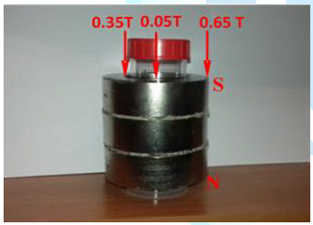Figure 1: Neodymium ring magnets applied for the surfactants solution treatment and the magnetic field strength on the edges and center of the magnet. The treated sample is placed in the magnet.

Results and Discussion

The results presented in Figure 2 show how the time-span of the MF treatment influences the surface tension of the solutions. In the case of SDS solution an increasing effect is observed with increasing treatment time but in the case of DoTAB such dependence is not straight. However, it needs further systematic investigations which will be the subject of a paper to follow. For present studies the 60 min MF treatment time have been selected.

The mean results of the subsequent measurements of surface tension of MF treated and untreated SDS solution samples are presented in Figure 3 for 0.001 M, 0.005 M and 0.01 M solutions. The surface tension of the magnetically treated solutions was measured immediately after the solution taking off the magnet. It usually took 3-5 min. As can be seen MF decreases surface tension of the solutions by 5.69 mN/m, 1.54 mN/m and 0.51 mN/m, respectively. It is clearly seen that with increasing the SDS solution concentration MF effect is smaller. It should be also stressed that the 0.01M concentration is a little larger than the CMC of SDS which amounts 0.0082 M.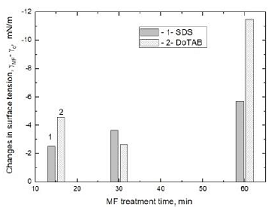Figure 2: Differences between surface tension of 10-3 M SDS and DoTAB samples MF-treated for different time and that MF-untreated, respectively.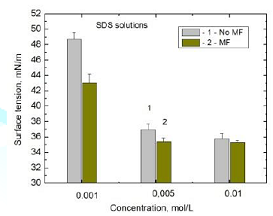Figure 3: Mean surface tension values of SDS 0.001 M, 0.005 M, and 0.01 M solutions: 1-MF-untreated and 2-60 min MF-treated samples.

The results of MF effects on DoTAB solution surface tension are shown in Figure 4. Comparing the results with those of Figure 3 the MF effect on the surface tension of this surfactant is much larger than that on SDS solutions. The surface tension of 0.001 M DoTAB solution decreased by 11.48 mN/m while the surface tension of 0.001 M SDS has decreased by 5.69 mN/m only. Similarly, in the case of 0.005M solutions the decrease in the surface tension is 5.72 mN/m and 1.54 mN/m, respectively. Moreover, the surface tension of 0.01M DaTAB solution after MF treatment increased by 5.95 mN/m and that of 0.05 M decreased by 0.85 ± 0.07 mN/m only. The 0.01M concentration is very close to CMC of DoTAB (0.011M) and 0.05M is significantly larger than CMC. The same values of surface tension of the untreated DoTAB solutions were reported by Acosta et al. . To better depict the MF effects on the surface tension of these two surfactants solutions in Figure 5 are shown the surface tension changes for all investigated concentrations. As it can be seen if the concentration is lower than the respective CMC, the surface tension of both surfactants is lowered by the MF action and a greater surface tension decrease is observed for cationic DoTAB than anionic SDS solutions. However, an increase in the surface tension (5.9 mN/m) of this surfactant solution occurs if the concentration (0.01M) is practically equal to its CMC (0.0011 M). If the concentrations of both surfactants are greater than their CMC the MF effects are very small, where the changes of surface tension are less than 1 mN/m (Figures 3-5).

The above results were elaborated statistically using the two-tailed t-Student test at 5% significance level. The results are collected in Table 1 where it can be seen that all the differences between MF treated and untreated solutions of the surfactants are statistically significant (p<<α). The mean values of the surface tension for each concentration were calculated from 5-8 individual experiments in which 10-15 times the surface tension was measured using the ring method.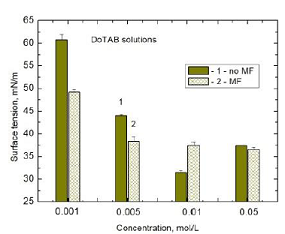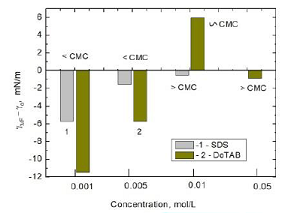Figure 5: Differences between surface tension of 60 min MF-treated and MF-untreated of SDS and DoTAB solutions of various concentrations below and above their CMC, respectively.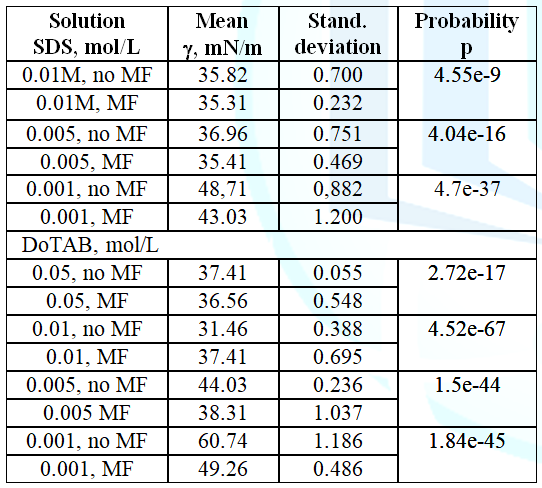Both surfactants possess the same chain-lenth containing 12 carbon atoms. However, their polar (ionic) heads are completely different, i.e. -OSO3H+ and -N+(CH3)3Br-

First of all the size of the groups is different, which is 0.17 nm2 for the SDS and 0.54 nm2 for the hard-core area of DoTAB. These values were established from the bond lengths, bond angles, and atomic volumes via a molecular model of the headgroup . Also the distance from the hydrophobic core surface to the centre of counterion location is 0.545 nm and 0.345 nm, respectively . Thus, the Na+ counterions are located at a larger distance from the C12-O-SO3- headgroup than Br- from the C12-N+(CH3)3. Moreover, the SDS molecules oxygen atoms can form hydrogen bonds with water molecules, which are not the case for DoTAB whose head is more hydrophobic because of presence of three methyl -CH3 groups and only a weak NH hydrogen bond can be formed . Because -N+(CH3)3 group is over 3 times larger than the sulfate, therefore it can be expected that less DoTAB groups (molecules) than those of SDS occupy the same surface area of the solution. Moreover, the Br- counterions are located closer to the heads , hence at the solution surface the interactions between water molecule and head group of DoTAB is much weaker than in the case of SDS.

Generally, the decrease in surface tension can be interpreted as due to an increase in the surface concentration of surfactant. This conclusion results from the Gibbs adsorption equation relating the surface excess amount of a surfactant with its bulk concentration and surface tension.

For Equation 1 refer PDF

From Equation 1 results that if a surfactant surface tension decreases with increasing its bulk concentration then the surface excess amount increases, but only below the CMC when in the surface adsorption layer maximum adsorption and packing of the molecules is not achieved yet. Assuming this interpretation, it would mean that the MF effect relies on the increasing excess of SDS or DoTAB molecules in the surface layer. Abandoning at this moment possible mechanism of the MF force action, the increase in surface excess concentration can be easily understood if the solution concentration is lower than CMC of SDS or DoTAB, respectively (Figures 3-5). Actually, also the small decrease in surface tension of the solution whose concentration is greater than CMC can be considered as due to some small changes in the density and structure of the adsorbed surface layer. Thus, in the case of 0.01 M SDS, this concentration is only slightly larger than the CMC by 0.0018 M (0.01 M-0.0082 M). Therefore there can be still some room in the surface layer for a closer SDS molecules packing caused by MF force and hence the observed small decrease in the surface tension. In the case of DoTAB after 60 min MF treatment the surface tension of 0.05 M decreases by 0.85 mN/m only. However, this small difference appeared to be statistical significant (Table 1). On the other hand the surface activity of surfactants can be described by Sprow and Prausnitz equation .

For Equation 2 refer PDF

Analogical equation can be written for water molecules in the surface layer.

For Equation 3 refer PDF

In Equations 2 and 3 activity a of the components are defined in the symmetrical system, i.e. aw, as ®1 if xw, xs ®1, respectively. Zdziennicka et al.  using Eq.3 and assuming that in diluted surfactant solutions its activity in the bulk phase is small and hence that of water is close to unity calculated activity of water in the surface layer from Eq.4 which results from Eq.3 if it is assumed that awB ®1.

For Equation 4 refer PDF

Where meaning of the symbols are the same as that in Equations 2 and 3.

In the calculations they  used for water gw = 72.8 mN/m at 293K and the molar surface area for water equal to 0.6023‧105 m2/mol. Then, because+= 1 and the adsorbed film pressure π is expressed as :

For Equation 5 refer PDF

For Equation 6 refer PDF

In the concentration range C below CMC of given surfactant (i.e. the range where the surface tension of the solution gLV vs. C decreases linearly) the calculated from Equation 6 gs values appeared to be constant (31.8 ± 0.3 mN/m) for the all 11 studied surfactants, among others SDS, CTAB and dodecyl dimethylethyl ammonium bromide . Hence they concluded that the maximum reduction of water surface tension equals to 72.8-31.8 = 41.0 mN/m. The value of gs= 31.8 mN/m lies between the surface free energy of polyethylene crystallized in air (36 mJ/m2)  and the surface tension of liquid hexadecane (27.5 mN/m). Generally, the surface free energy of solid hydrocarbons is much higher if the hydrocarbon chains are parallel than normal to surface. This is because of a lower surface concentration of -CH3 than -CH2- groups, i.e. 0.19-0.25 nm2 and 0.05-0.057 nm2, respectively . However, -CH3 interaction is 82% higher than -CH2- . In the above calculation the authors  assumed the cross-sectional area for water molecule equal to 0.1 nm2. Such value was determined for adsorbed water molecules . The van der Waals diameter (an imaginary hard sphere representing the distance of closest approach) of water molecule is much smaller 0.282-0.32 nm and therefore its cross-sectional area is 0.62-0.80 nm2 . It means that the surface area per water molecule in the surface layer can be squeezed. The above mentioned maximum possible reduction of the surface tension of water (41.0 mN/m) is smaller than the surface tension values of our MF treated 10-3 M SDS and DoTAB solutions. However, if the concentration of SDS is higher than 10-3 M the surface tension of both MF treated and an untreated solution is 5-6 mN/m smaller than 41.0 mN/m (Figure 3, Table 1). But such concentration is already behind the linear change of surface tension versus concentration. The same is true for DoTAB solutions but for 0.01 M and 0.05 M solutions (Figure 4, Table 1). The calculated from Equation 6 values of gs for 10-3 M SDS amount to 18.8 mN/m and 16.3 mN/m for untreated and MF treated solutions, respectively, and the values for 0.001 M DoTAB solution equal to 25.4 mN/m and 17.4 mN/m, respectively. Thus calculated gs values for given solution can be interpreted as free energy of the surface layer built up solely of the surfactant molecules. The layer structure and the molecules orientation determine its free energy and changes similarly as that of the n-alkanes series from n-pentane to n-hexadecane whose surface tension changes from 15.8 mN/m to 27.5 mN/m, respectively.

As discussed above, bigger decrease in the surface tension of cationic than anionic surfactant solutions caused by MF is probably due to the presence of three -CH3 groups present in the DoTAB head group. The interaction potential of this group is greater than –CH2– although the surface density of the groups is lowers . Hence the decrease in surface tension of MF treated solutions may result from some reorientation of the adsorbed surfactant molecules and changes in the surface layer structure. The different properties of -N+(CH3)3 and -O-SO3- groups reflected also in water rate evaporation from these surfactant solutions. The former caused easier evaporation of water while the later hindered it . Additionally, the increased water evaporation from MF treated DoTAB solution might be also due to weakening of Van der Waals interactions and hydrogen bonds of the intra-clusters [2,33]. On the other hand, formation of hydrogen bonds of water molecules with the oxygen atoms from -OSO3- groups hinders water evaporation . Van Oss and Constanzo  reported for SDS immersed in water 23.8 mN/m for the surface tension of tails and 46 mN/m for the electron-donor parameterof -SO4 head, which is responsible for the hydrogen bonds formation. From the surface tension values of SDS solutions (Figure 3 and Figure 4) results that theprincipally determines the surface tension. As for the increase in surface tension of MF treated 10-2 M DoTAB solution (Figure 3 and Figure 4), which is very close to the CMC but a little below, it can be interpreted as the restructuring of the almost fully packed surface layer. From the Gibbs adsorption equation (Equation 1) it can be concluded that MF enhances formation of micelles in the bulk solution and hence reduces the effective DoTAB bulk activity which appears in a small increase of the solution surface tension. However, the observed decrease in the DoTAB surface tension of the solutions in the rest cases leads to the conclusion that MF increases amount of the adsorbed surfactant molecules in the surface layer and/or causes the layer restructuration (the molecules reorientation) if below CMC and practically has no effect on the solutions at a higher concentration than CMC.

Trying to understand the observed magnetic field effects on the surface tension changes of ionic surfactant solutions the Lorentz force action can be considered.

For Equation 7 refer PDF

The first term represents the electric force acting on a moving charge v and the second term expresses the magnetic force whose direction is perpendicular to both the velocity of the charge and the magnetic field. The magnetic force action depends on the charge q and the magnitude of so called cross product of v × B, i.e. the velocity and flux density vectors where the relative directions of these two vectors are taken into account. Depending on the angle ϕ between v and B, the magnitude of the force equals qvB sin ϕ. If the angle ϕ = 90o, i.e. v is perpendicular to B, the particle trajectory is circular with a radius of r = mv/qB. If the angle ϕ is less than 90°, the particle will move along a helix having the axis parallel to the field lines. Finally, if ϕ = 0o there will be no magnetic force acting on the particle and it will continue moving along the field lines. In an electrolyte solution the electric field density E is zero and only magnetic force of Equation 7 acts. Among others Silva et al.  considered the Lorentz force to be responsible for the observed MF effects which influenced the ion polarization, especially bivalent cations which are hydrated more strongly than the anions. The ions remained orientated up to two days (the memory effect) at the gas nanobubbles dispersed in the solution. In consequence the precipitating particles of calcium carbonate were smaller than those from the untreated solutions. Also some changes in their crystalline structure were observed . Taking magnitude of the parameters as: v @ 0.992 m/s (evaluated experimentally), q = 3.2 ‧10-19 C (divalent cation), E = 0 (electrolyte solution) and B = 1T, the Lorentz force amounted to 3.17‧10-19 N. Because the ion mass is 10-25-10-26 kg, therefore the acceleration (F/m) can be as large as 106-107 m/s2, and it would cause the ion polarization. Moreover, this was confirmed by experiments conducted under the quiescent conditions where no changes in the liquid viscosity or particles settling rates were observed . In our experiments the MF in the ring magnet changes radially from the top inner edge to its center from 0.347 T to 0.053 T which occurs on the distance of 19 mm (Figure 1). The MF derivative ¶B/¶x on the surfactant sample surface level equals to 43.18 T/m and 7.91 T/m, respectively. Hence MF gradient changes from 14.96 T2/m to 0.42 T2/m, respectively (Figures 8 and 9 ). Assuming that during the samples stirring every 15 min the surfactant ions in the solution move ca. 0.5 m/s and some of the ions cross perpendicularly the field lines. Then the Lorentz force F = qvB for monovalent ion amounts to (1.6×10-19 C × 0.5 m/s × 0.347 T) = 0.278×10-19 N. Hence the acceleration force F/m imposed on the dodecylsufate ion C12-O-SO3-(4.406×10-25 kg/ion) amounts to 6.3 × 104 m/s2 while that acting on C12-N(CH3)3+ (3.79 ×10-25 kg/ion) amounts to 7.3 × 104 m/s2. The force at the magnet center is ca. 6.5 times lower than that at the edge. Although calculated here acceleration force values are much lower than those calculated by Silva et al  in the 1T magnetic field, it seems that they are still can influence the surfactant surface monolayer formation and structure, which appears in the observed changes of the solutions surface tension. Moreover, the observed MF effects can be also considered as a result of the local increase in the thermodynamic potential. Such approach was described in detail in the papers published by Cefalas et al. [8,9] who explained why even in a weak external magnetic field (0.05 T) aragonite precipitates instead of calcite, nevertheless that the later has lower ground electronic state by 28 eV. They explained this phenomenon on the basis of a macroscopic antisymmetric coherent state. Such state can be induced by an external MF acting on an ensemble of water two level molecular rotors (the water coherent state). In the case of a conductive liquid, even in absence of a static MF, during the flow there are present electromagnetic fluctuations and spontaneous magnetic field. Quantum mechanics predicts that external magnetic field can amplify magnetic fluctuations in the bulk liquid by exchanging the energy through an angular momentum of water molecular rotors, as well as by such momentum of the macroscopic turbulent flow. Thus, the energy transfer occurs via the angular momenta of water molecules, the flow and the magnetic field. From the theoretical calculation results that the received energy increases if the MF is in resonance with the rotational frequencies of molecular rotors and low frequencies of the turbulent flow . Moreover, according to these authors [8,9] such amplified state will last for a longer time because of “the forbidden nature of transition between the anti-symmetric and the symmetric state” . This explains precipitation of CaCO3 as aragonite and also so called memory effect in water which was many times reported in the papers. Later Coey  proposed a theory explaining the MF effects which considered the field gradient as more important than the MF strength itself. It is based on a non-classical nucleation mechanism which takes into account the presence of stable pre-nucleation clusters in calcium carbonate solutions. Next this theory has been successfully verified by Sammer et al. . Relating these approaches to the observed changes in the surface tension of the surfactant solutions it can be easier understood the surface tension changes as due to the MF energy gained by the surfactant ions, the state of which lasts sufficiently long to measure the changes after several minutes the MF had ceased. It should be also mentioned that in the previously published paper  we had observed decrease in the surface tension of pure water by 2.1 mN/m after 60 min of the same MF treatment. Moreover, after 60 min since the field removal the surface tension was still by 1 mN/m smaller than that of the untreated sample.

Conclusions

Static Magnetic Field (MF) affects surface tension of anionic and cationic surfactant solutions. A bigger effect has been found for cationic than anionic surfactant solutions. The MF effects are very small in the solutions whose concentration is higher than CMC of the given surfactant. The MF effects can result both from the Lorentz force and the local increase in the thermodynamic potential. It can be induced by an external MF acting on an ensemble of water two level molecular rotors and can be explained on the basis of a macroscopic antisymmetric coherent state. The local increase in the MF gradient can cause the effects too. The presented results are somewhat preliminary and the above calculations and hypotheses have to be verified by systematic studies. So far no MF effects dealing with the surfactant solutions were published and there is no data for any comparison. Therefore more experiments are needed to better understand the observed changes and the above presented results should be treated as preliminary ones.

Acknowledgements

This work was supported by National Centre of Science, grant 2016/21/B/ST4/00987, which is greatly appreciated. We are grateful to Ms. Weronika Głąb for the surface tension measurements and MSc Michał Chodowski for statistical calculations.

References

1.        Toledo EJL, Ramalho TC and Magriotis ZM. Influence of magnetic field on physical-chemical properties of the liquid water: Insights from experimental and theoretical models (2008) J Molecular Sci 888: 409-415. https://doi.org/10.1016/j.molstruc.2008.01.010

2.        Zhou KX, Guiwu L, Zhou QC and Song JH. Monte Carlo simulation of liquid water in a magnetic field (2000) J Appl Phys 88: 1802-1805. https://doi.org/10.1063/1.1305324

3.        Chang KT and Weng CI. The effect of an external magnetic field on the structure of liquid water using molecular dynamic simulation (2006) J Appl Phys 100: 043917. https://doi.org/10.1063/1.2335971

4.        Cai RH, Yang JH and ZhuW. The effects of magnetic fields on water molecular hydrogen bonds (2009) J Mol Struct 938: 15-19. https://doi.org/10.1016/j.molstruc.2009.08.037

5.        Coey JMD. Magnetic water treatment-how might it work? (2012) Philos Mag 92: 3857-3865. https://doi.org/10.1080/14786435.2012.685968

6.        Demichelis R, Raiteri P, Gale JD, Quigley D and Gebauer D. Stable prenucleation mineral clusters are liquid-like ionic polymers (2011) Nat Comm 2: 590-598. http://doi.org/10.1038/ncomms1604

7.        Sammer M, Kamp C, Paulitsch-Fuchs AH, Wexler AD, Cees J N, et al. Strong gradients in weak magnetic fields induce DOLLOP formation in tap water (2016)Water 8: 79. https://doi.org/10.3390/w8030079

8.        Cefalas AC, Kobe S, Dražic G, Sarantopoulou E, Kollia Z, et al. Nanocrystallization of CaCO3 at solid/liquid interfaces in magnetic field: a quantum approach (2008) Appl Surf Sci 254: 6715-6724. https://doi.org/10.1016/j.apsusc.2008.04.056

9.        Cefalas AC, Sarantopoulou E, Kollia Z, Riziotis C, Dražic G, et al. Magnetic field trapping in coherent antisymmetric states of liquid water molecular rotors (2010) J Comput Theor Nanosci 7: 1800-1805. https://doi.org/10.1166/jctn.2010.1544

10.     Chibowski E and Szcześ A. Magnetic water treatment - A review of the latest approaches (2018), Chemosphere 203: 54-67. https://doi.org/10.1016/j.chemosphere.2018.03.160

11.     Nakagawa J, Hirota N, Kitazawa K and Shoda M. Magnetic field enhancement of water vaporization (1999) J Appl Phys 86: 2923-2925. https://doi.org/10.1063/1.371144

12.     Kitazawa K, Ikezoe Y, Uetake H and Hirota N. Magnetic field effects on water, air and powders (2001) Physica B 294-295: 709-714. https://doi.org/10.1016/S0921-4526(00)00749-3

13.     Holysz L, Szczes A and Chibowski E. Effects of a static magnetic field on water and electrolyte solutions (2007) J Colloid Interface Sci 316: 996-1002. https://doi.org/10.1016/j.jcis.2007.08.026

14.     Szcześ A, Chibowski E, Holysz L and Rafalski P. Effects of static magnetic field on water at kinetic condition (2011) Chem Eng Process 5: 124-127. https://doi.org/10.1016/j.cep.2010.12.005

15.    Rashid FL, Hassan NM, Jafar AM and Hashim A. Increasing water evaporation rate by magnetic field (2013) Int Sci Invest J 2: 61-68.

16.     Guo Y-Z, Yin D-C, Cao H-L, Shi J-Y, Zhang C-Y et al. Evaporation rate of water as a function of a magnetic field and field gradient (2012) Int J Mol Sci 13: 16916-16928. https://dx.doi.org/10.3390%2Fijms131216916

17.     Seyfi A, Afzalzadeha R and Hajnorouzi A. Increase in water evaporation rate with increase in static magnetic field perpendicular to water-air interface (2017) Chem Eng Process 120: 195-200. https://doi.org/10.1016/j.cep.2017.06.009

18.     Amor HB, Elaoud A, Salah NB and Elmoueddeb K. Effect of Magnetic Treatment on Surface Tension and Water Evaporation (2017) Intern J Advan Ind Engin 5: 119-124. http://dx.doi.org/10.14741/Ijae/5.3.4

19.     Mang Y, Wei H and Li Z. Effect of magnetic field on physical properties of water (2018) Results Phys 8: 262-267. https://doi.org/10.1016/j.rinp.2017.12.022

20.     Chibowski E, Szcześ A and Hołysz L. Influence of magnetic field on evaporation rate and surface tension of water (2018) Colloids Interfaces 2: 68. https://doi.org/10.3390/colloids2040068

21.     Ivanković T and Hrenović J. Surfactants in the environment (2010) J Surfactants Environment 61: 95-110. https://doi.org/10.2478/10004-1254-61-2010-1943

22.     Olkowska E, Polkowska Z and Namiesnik J. Analytics of surfactants in the environment: problems and challenges (2011) Chem Rev 111: 5667-5700. https://doi.org/10.1021/cr100107g

23.     Chibowski E and Szcześ A. Magnetic field effects on aqueous anionic and cationic surfactant solutions. Part I: Water evaporation, submitted for publication.

24.     Acosta EJ, Mesbah A and Tsui T. Surface activity of mixtures of dodecyltrimethyl ammonium bromide with sodium perfluorooctanoate and sodium octanoate (2006) J Surfactants Detergents 9: 367-376. https://doi.org/10.1007/s11743-006-5015-y

25.     Fujiwara F, Reeves LW, Suzuki M and Vanin JA. Studies of lyotropic liquid crystals that align in magnetic fields (1979) In: Proceedings of Colloids and Surface Science Symposium, University of Tennessee, Solution chemistry of surfactants, USA 1: 63-78.

26.     Nagarajan R and Wang C-C. Theory of surfactant aggregation in water/ethylene glycol mixed solvents (2000) Langmuir 16: 5242-5251. https://doi.org/10.1021/la9910780

27.     Sprow FB and Prausnitz JM. Surface thermodynamics of liquid mixtures (1992) Can J ChemEng 6: 25-28. https://doi.org/10.1002/cjce.5450450106

28.     Zdziennicka A, Szymczyk K, Krawczyk J and Jańczuk B. Activity and thermodynamic parameters of some surfactants adsorption at the water-air interface (2012) Fluid Phase Equilibria 318: 25-33. https://doi.org/10.1016/j.fluid.2012.01.014

29.     Chattoraj DK, Halder E, Das KP and Mitra A. Surface activity coefficients of spread monolayers of behenic acid salts at air–water interface (2006) Adv Colloid Interface Sci 123-126: 151-161. https://doi.org/10.1016/j.cis.2006.05.002

30.     Fowkes FM. Calculation of work of adhesion by pair potential summation (Ed) Fowkes FM (1969) Acad Press, UK, 151-163. https://doi.org/10.1016/B978-0-12-395594-4.50021-X

31.     Van Olphen H. Determination of surface areas of clays- evaluation of methods (Eds) Everett DH and Ottewill RH (1970) Surface Area Determination: Proceedings of the International Symposium on Surface Area Determination Held at University of Bristol, UK 255-272. https://doi.org/10.1016/B978-0-408-70077-1.50027-0

32.     Chaplin M. Water Structure and Science

33.     Krems RV. Breaking van der Waals molecules with magnetic fields (2004) Phys Rev Lett 93: 013201. https://doi.org/10.1103/PhysRevLett.93.013201

34.     Van Oss CJ and Costanzo PM. Adhesion of anionic surfactants to polymer surfaces and low energy materials (1992) J Adhesion Sci Technol 6: 477-487. https://doi.org/10.1163/156856192X00809

35.     Silva B, Queiroz Neto JC and Petria DFS. The effect of magnetic field on ion hydration and sulfate scale formation (2015) Colloid Surf A 465: 175-183. https://doi.org/10.1016/j.colsurfa.2014.10.054

*Corresponding author

Emil Chibowski, Professor, Department of Physical Chemistry-Interfacial Phenomena, Faculty of Chemistry, Maria Curie-Skłodowska University, 20-031 Lublin, Poland, E-mail: emil.chibowski@umcs.pl

Citation

Chibowski E, Szcześ A and Hołysz L. Magnetic field effects on aqueous anionic and cationic surfactant solutions part II: surface tension (2019) Edelweiss Chem Sci J 2: 1-6

Keywords

Magnetic field effects, Anionic and cationic surfactant, Surface tension changes